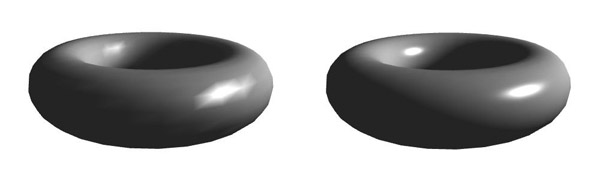Prev: Dir Lights per Vertex II Next: Point Lights

The previous sections described Gouraud shading, where a colour is computed per vertex, and the fragments get their colour values through interpolation.

Phong, the same person behind the specular equation, proposed that, instead of interpolating colours, we should interpolate normals (and other relevant data) and compute the actual colour per fragment. This is known as the Phong shading model. While not perfect, this is certainly an improvement quality wise as we’ll see.

The vertex shader is only going to prepare the data that needs to be interpolated, and the fragment shader will perform the colour computation based on per fragment values.

To compute the colour the fragment shader will need to receive the following per fragment data:

• normal
• eye vector

The vertex shader must compute/transform these vectors per vertex, so that they get interpolated and passed on to the fragment shader. To compute the eye vector we must compute a vector from the point being lit to the camera, in camera space.$Eye = Cam - point$

Where point is the position in camera space. To compute point we multiply the view model matrix by the vertex position. We also know that, in camera space, the camera is located at the origin, so the vector is simply:$Eye = - m\_viewModel \times position$

The new vertex shader is:

#version 330

layout (std140) uniform Matrices {
mat4 m_pvm;
mat4 m_viewModel;
mat3 m_normal;
};

in vec4 position;	// local space
in vec3 normal;		// local space

// the data to be sent to the fragment shader
out Data {
vec3 normal;
vec4 eye;
} DataOut;

void main () {

DataOut.normal = normalize(m_normal * normal);
DataOut.eye = -(m_viewModel * position);

gl_Position = m_pvm * position;
}

Note that the light’s direction is no longer dealt with in the vertex shader. This is because the direction is constant for all points (after all this is a directional light!). Being constant means that we know its value for each fragment, without having to compute it per vertex and interpolate it. Besides, it would yield exactly the same value after interpolation. Hence, we can feed it directly to the fragment shader.

Most of the work will be performed in the fragment shader. This shader receives the interpolated normal and eye vectors, and computes the colour. The computation itself is identical to the previous section.

#version 330

layout (std140) uniform Materials {
vec4 diffuse;
vec4 ambient;
vec4 specular;
float shininess;
};

layout (std140) uniform Lights {
vec3 l_dir;	   // camera space
};

in Data {
vec3 normal;
vec4 eye;
} DataIn;

out vec4 colorOut;

void main() {

// set the specular term to black
vec4 spec = vec4(0.0);

// normalize both input vectors
vec3 n = normalize(DataIn.normal);
vec3 e = normalize(vec3(DataIn.eye));

float intensity = max(dot(n,l_dir), 0.0);

// if the vertex is lit compute the specular color
if (intensity > 0.0) {
// compute the half vector
vec3 h = normalize(l_dir + e);
// compute the specular term into spec
float intSpec = max(dot(h,n), 0.0);
spec = specular * pow(intSpec,shininess);
}
colorOut = max(intensity *  diffuse + spec, ambient);
}

The result is much nicer than in the previous section. On the left we have a directional light with colour computed per vertex, and on the right the colour is computed per fragment.Prev: Dir Lights per Vertex II Next: Point Lights

This site uses Akismet to reduce spam. Learn how your comment data is processed.